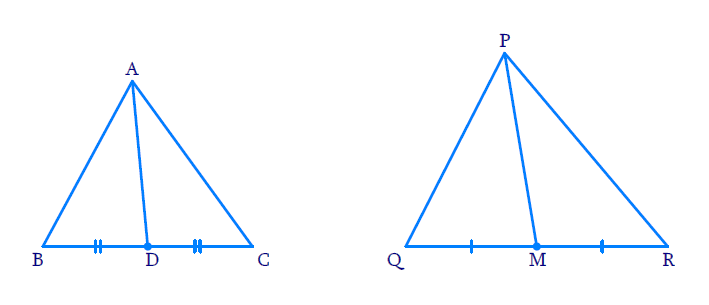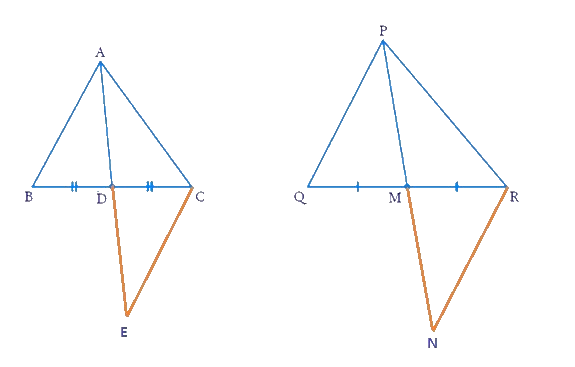# Ex.6.3 Q14 Triangles Solution - NCERT Maths Class 10

## Question

Sides $$AB$$ and $$AC$$ and median $$AD$$ of a $$\Delta ABC$$ are respectively proportional to sides $$PQ$$ and $$PR$$ and median $$PM$$ of another $$\Delta PQR.$$

Show that $$\Delta ABC\text{ }\sim{\ }\text{ }\Delta PQR$$ .

DiagramVideo Solution
Triangles
Ex 6.3 | Question 14

## Text Solution

Reasoning:

As we know if one angle of a triangle is equal to one angle of the other triangle and the sides including these angles are proportional, then the two triangles are similar.

This criterion is referred to as the $$\rm SAS$$ (Side–Angle–Side) similarity criterion for two triangles.

Steps:Produce $$AD$$  to $$E$$ so that $$AD = DE.$$ Join $$CE$$ Similarly, produce $$PM$$ to $$N$$ such that $$PM = MN$$, and Join $$RN$$.

In $$\Delta ABD$$  and $$\Delta CDE$$

\begin{align}&\quad \;\, AD = DE\\ \text{[By } & \text{Construction]}\\\\&\quad \;\, BD = DC\\ [\text{AP } & \text{is the median}] \\\\& \angle ADB = \angle CDE \\ [ \text{Vertically }&\text{opposite angles}] \\\\\therefore &\Delta ABD \cong \Delta CDE \\ [\text{By SAS } & \text{Criterion of congruence}] \\\\ \Rightarrow &\quad \;\; AB = CE \;[ CPCT] \dots \text{(i)} \end{align}

Also, in $$\Delta PQM$$ and $$\Delta MNR$$

\begin{align}PM &= MN \\ \text{[By }&\text{Construction]}\\\\ QM &= MR \\ \text{[PM is } &\text{the median]} \\\\ \angle PMQ &= \angle NMR \\ \text{[Vertically }& \text{opposite angles]} \\\\ \therefore \Delta PQM &= \Delta MNR \\ \text{By SAS }& \text{criterion of congruence}\\\\ \Rightarrow PQ &= RN [CPCT] \text{... (ii)}\end{align}

Now,

\begin{align} &\frac{{AB}}{{PQ}} = \frac{{AC}}{{PR}} = \frac{{AD}}{{PM}} \;\; \left[ {{\rm{Given}}} \right]\\ &\Rightarrow \frac{{CE}}{{RN}} = \frac{{AC}}{{PR}} = \frac{{AD}}{{PM}}\\ &\qquad [\text{From (i)} \text{ and (ii)} ] \\\\& \Rightarrow \frac{{CE}}{{RN}} = \frac{{AC}}{{PR}} = \frac{{2AD}}{{2PM}}\\ &\Rightarrow \frac{{CE}}{{RN}} = \frac{{AC}}{{PR}} =\frac{{AE}}{{PN}} \\ &[ 2AD = AE\;{\text{ and }}\; 2PM = PN ] \\\\ &\therefore \Delta ACE\sim\Delta PRN \\& [ \text{By SSS} \text{ Similarity} \text{ Criterion}]\end{align}

Therefore,

$\angle CAE = \angle RPN$

Similarly,

$\angle BAE = \angle QPN$

Therefore,

\begin{align} \angle CAE \!\!+\! \angle BAE &\!=\! \angle RPN \!\! +\!\! \angle QPN \\ \Rightarrow \angle BAC &= \angle QPR\\ \Rightarrow \angle A &= \angle P....{\rm{(iii)}}\end{align}

Now, in \begin{align}\Delta ABC\;{\text{ and }}\;\Delta PQR\end{align}

\begin{align}&\frac{{AB}}{{PQ}} = \frac{{AC}}{{PR}}\\&\;\;\angle A = \angle P\quad \dots\left[ {{\rm{from (iii)}}} \right]\\\\&\therefore \Delta ABC\sim\Delta PQR \\ & [ \text{By SAS} \text{ similarity} \text{ criterion} ] \end{align}

Learn from the best math teachers and top your exams

• Live one on one classroom and doubt clearing
• Practice worksheets in and after class for conceptual clarity
• Personalized curriculum to keep up with school